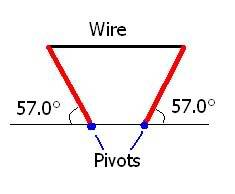# Frequency and Wavelength Problem

## Homework Statement

A 5.00-m, 0.732-kg wire is used to support two uniform 235-N posts of equal length (see figure). Assume that the wire is essentially horizontal and that the speed of sound is 344 m/s. A strong wind is blowing, causing the wire to vibrate in its 7th overtone. What are the frequency and wavelength of the sound this wire produces?## Homework Equations

at 7th overtone: L= 8(lamda/2)

## The Attempt at a Solution

lamda = (2/8)L = (2/8)(5) = (5/4) m

Is my wavelength correct?
I'm not sure of which equation to use to find the frequency.

frequency{n}=(pth overtone+1)xvelocity of sound in air
------------------------------------------
2xlength of wire

therefore n= (7+1) x 344
--------------- =6880Hz
2x5

alphysicist
Homework Helper
A commonly useful relationship between wavelength and frequency in a wave is

speed of wave = frequency X wavelength

Once you have the wavelength of the wire's vibration, you can find the frequency once you know the speed of a wave in this stretched wire. There is a standard textbook formula for the speed of a wave in a stretched string involving the linear mass density (which is mass/length if the wire is uniform) and the tension in the wire, so first you'll need to find the tension by treating the wire and posts as an equilibrium problem.

Finally, if I'm reading the question correctly, you need to find the wavelength and frequency of the sound wave in the air. In other words how is the wavelength and frequency of the wire's vibration related to the wavelength and frequency of the air's vibration? What will be the same, and how can you find the other?

------------------------------

Hi Vishal60,

I don't believe your approach is correct; since all of the strings in a guitar have the same vibration length, your equation would predict that all of the strings have the same frequency and therefore the same sound for their seventh overtones.